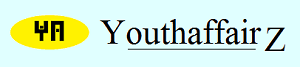July 2016

# Solution

Let Rohan's speed be "r" km/hr and Amit's speed be "a" km/hr. Since Rohan's speed is "r", he covers 2 kilometres in 2/r hours. Similarly, Amit covers 2 kilometres in 2/a hours.

Amit takes two minutes longer. In terms of hours, Amit takes 2/60 or 1/30 hours longer to complete the race. Therefore, 2/a - 2/r = 1/30.

So, (2r - 2a)/ar = 1/30;

2r - 2a = ar/30;

r - a = ar/60;

60(r - a) = ar.

Now, if Rohan had reduced his speed by 2 km/hr his speed would have been (r-2) km/hr and he would have covered 2 kilometres in 2/(r-2) hours. If Amit had increased his speed by 2 km/hr his speed would have been (a+2) km/hr and he would have covered 2 kilometres in 2/(a+2) hours. In this case, Amit would have won the race by two minutes. Amit wins the race implies he takes less time, so 2/(a+2) is less than 2/(r-2). So, in this case 2/(r-2) - 2/(a+2) = 1/30.

Therefore, [2(a+2) - 2(r-2)] / [(r-2)(a+2)] = 1/30;

So, 2(a+2) - 2(r-2) = [(r-2)(a+2)]/30;

So, 60(a+2) - 60(r-2) = (r-2) (a+2);

So, 60a + 120 - 60r + 120 = ra + 2r - 2a - 4;

So, 62a - 62r + 244 = ra;

But from the first case we have seen that 60(r - a) = ar.

Let's substitute; we get 62a - 62r + 244 = 60(r - a).

So, 62a - 62r + 244 = 60r - 60a;

So, 122a - 122r + 244 = 0.

Thus, 122 (a - r) = -244; or (a - r) = -2; or (-r + a) = -2; or -(r-a) = -2, or (r-a) = 2.

But, 60(r - a) = ar; so, 60 x 2 = ar; or ar = 120.

Now I know that (x - y)2 = x2 - 2xy + y2; thus, (r - a)2 = r2 - 2ra + a2. I can rewrite this as: (r - a)2 = r2 + 2ra + a2 -4ra.

So, (r - a)2 = (r+a)2 - 4ra.

So, 22 = (r+a)2 - 4(120) ..... because (r - a) = 2 and ar = 120.

So, 4 = (r+a)2 - 480; so, (r+a)2 = 484.

Therefore, r + a = +22 or -22. But we are dealing with speeds which cannot be negative. Therefore, r + a = 22.

Thus, we have (r-a) = 2 and (r+a) = 22; solving these two equations we get r = 12 and a = 10. Thus, Rohan's speed is 12 km/hr and Amit's speed is 10 km/hr. Did I do it right? I seem to have taken ages and ages to solve this! Is there any simple method to solve it?

• Union Public Service Commission - www.upsc.gov.in
• IIT-Kharagpur - www.iitkgp.ac.in
• Indian Statistical Institute - www.isical.ac.in
• Indian Institute of Technology Madras - www.iitm.ac.in
• Indian Institute of Management, Ahmedabad - www.iimahd.ernet.in
• Indian Institute of Mass Commission - www.iimc.nic.in
• IIT Bombay - www.iitb.ac.in